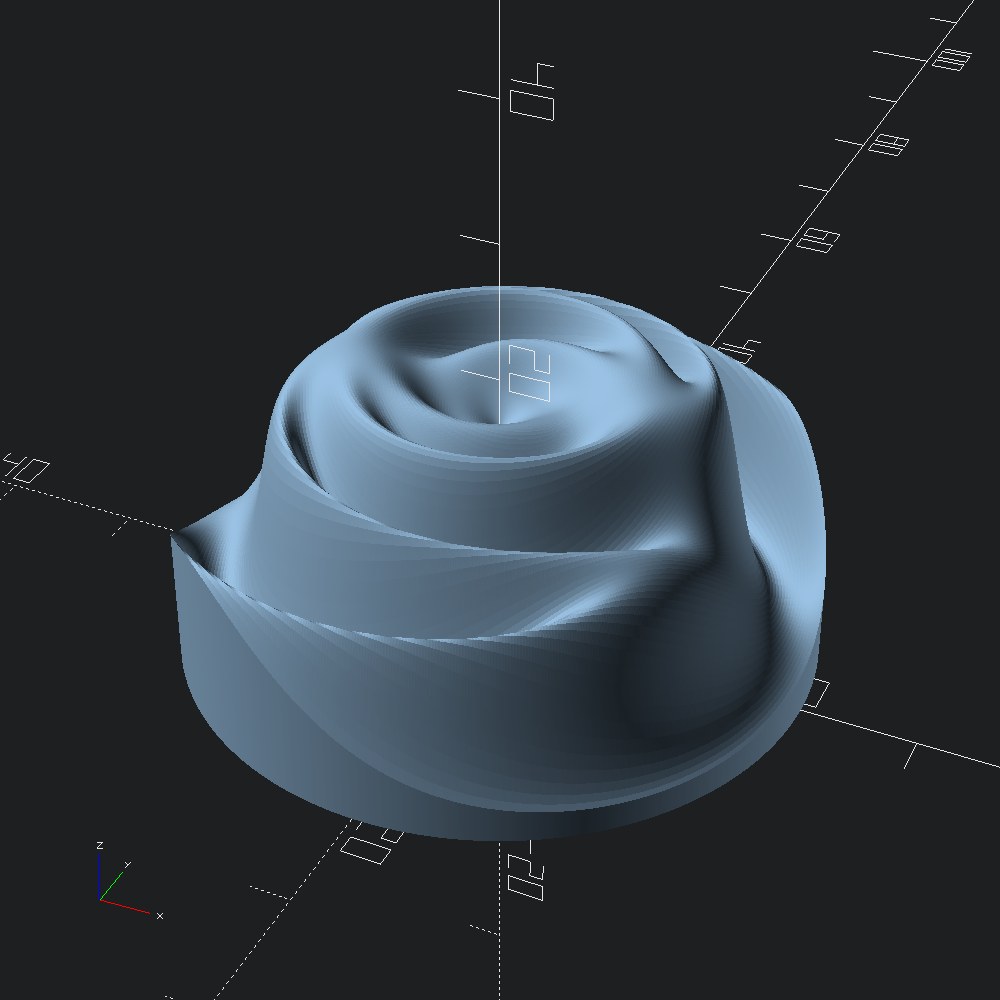# Polar Rose

``````open OCADml

Define a function that takes a radius `r` and angle `a`, and returns a z coordinate. This rose function is ported from the examples given in the plot function OpenSCAD library.

``````let rose ~r ~a =
let open Float in
let x =
pow
( (r *. cos a *. cos (r *. 8. *. pi /. 180.))
+. (r *. sin a *. sin (r *. 35. *. pi /. 180.)) )
2.
/. -300.
in
((15. +. (5. *. sin (r *. 10. *. pi /. 180.))) *. exp x) +. 1.``````

Starting from the origin, step outward radially with `r_step` increments up to a maximal radius of `max_r`. For each of these radii, `rose` will be evaluated with angles revolving around the z-axis, evaluating to the height off of the XY plane that that position should be.

``let mesh = Mesh.polar_plot ~r_step:0.2 ~max_r:22. rose``

As the generated mesh contains quite a large number of points due to the relatively low value of `r_step` (megabytes), we'll take advantage of the `include` trick provided by the optional `?incl` parameter to `Scad.to_file`. This will produce a pair of `.scad` scripts, one named `"incl_polar_rose.scad"`, and another named `"polar_rose.scad"` that simply includes it. By loading the later into OpenSCAD rather than the former, we can avoid the sluggishness that can result when the editor attempts to handle large files.

``let () = Scad.to_file ~incl:true "polar_rose.scad" (Scad.of_mesh mesh)``